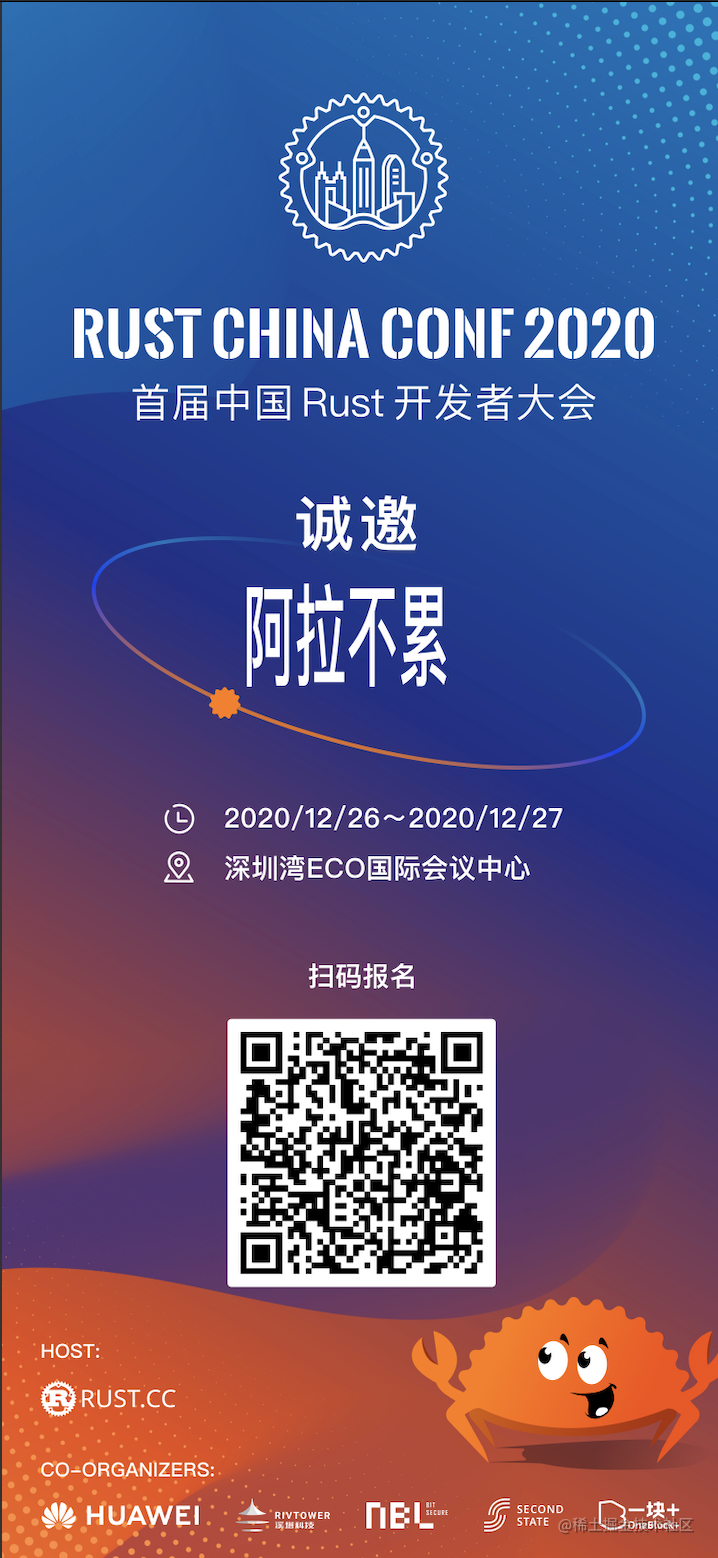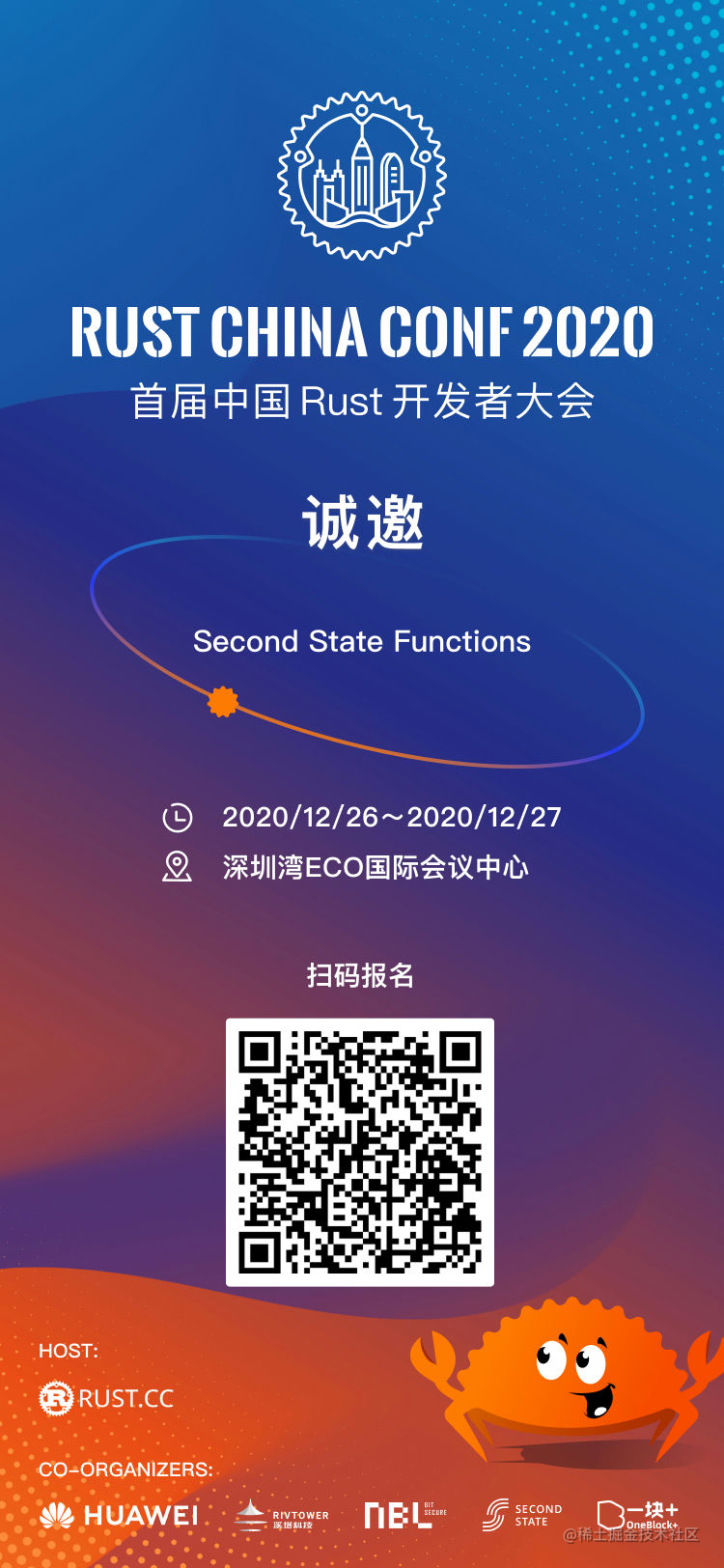# 🦀 详解一键生成邀请海报工具 | Rust 与 WebAssembly

• 设计确定好海报样式；
• 运营提供给设计名字；
• 设计根据名字一张一张输出海报；
• 运营再把海报一张一张发给参与者；

### 不就是改改参数吗？🤔

• 找到一个中英文通用的字体，没有任何版权问题
• 重新计算 watermark 在海报上的位置，居中放置
• watermark 的颜色与大小
``````#[wasm_bindgen]
pub fn watermark (img_buf: &[u8]) -> Vec<u8> {
// 读取输入的图片
let (w,h) = img.dimensions();
let scale = Scale {
x: w as f32 /10.0,
y: h as f32 /10.0,
};

// 准备 watermark 的字体
let font = Vec::from(include_bytes!("DejaVuSans.ttf") as &[u8]);
let font = Font::try_from_vec(font).unwrap();

// 在输入的图片画出 watermark
drawing::draw_text_mut(&mut img, image::Rgba([255u8, 255u8, 255u8, 255u8]), 0+(h/10),h/2, scale, &font, "Hello Second State");

// 编写并返回已经加好水印的照片
let mut buf = vec![];
img.write_to(&mut buf, image::ImageOutputFormat::Png).unwrap();
return buf;
}

` let font = Vec::from(include_bytes!("DejaVuSans.ttf") as &[u8]);` 在这里替换字体，用 `PingFang Bold.ttf` 替换原来的字体。

`PingFang Bold.ttf` 支持中英文，也与 Rust China Conf 的主 kv 字体一致，是个完美的选择。

` Rgba([255u8, 255u8, 255u8, 255u8]), 0+(h/10),h/2,` 在这里调整颜色的 RGB 值与位置，RGB 值也就是水印的颜色。根据海报的背景最终选定白色。再接下来就是通过调整 `0+(h/10),h/2,` 调整字体的位置了。### 改参数也是要技巧的 🧐

😭 😭 😭

``````const MAX_WIDTH : u32 = 349;
const MAX_HEIGHT : u32 = 80;
const MAX_FONT_SIZE : f32 = 125.0;
const FAR_LEFT : u32 = 200;
const FAR_TOP : u32 = 590;

`lib.rs` 先确定了输入文字的宽度与高度、基准字号、文字的左上角位置，这里可以理解成先规定了一个安全区域。

``````pub fn watermark (watermark_text: &str) -> Vec<u8> {
let width = write_to_crop(watermark_text);

let mut left = FAR_LEFT;
let mut top = FAR_TOP;
let mut font_size = MAX_FONT_SIZE;

`lib.rs` 里的 `watermark` 函数根据 `imagecrop` 里计算出的基准宽度，以及海报上允许放置文字的最大尺寸和相对位置，计算出能让文字居中显示的位置和字号。

``````if width < MAX_WIDTH {
left = FAR_LEFT + (MAX_WIDTH - width) / 2;
} else {
font_size = (MAX_WIDTH as f32) / (width as f32) * MAX_FONT_SIZE;
top = FAR_TOP + ((1.0 - (MAX_WIDTH as f32) / (width as f32)) * (MAX_HEIGHT as f32)) as u32;
}### 生成适合自己的海报，解放双手 👐

`template.png` 是模板海报，你可以替换成自己的海报。如果你的海报宽度与我们的海报宽度一样，且名字的位置也一样，那么就不用调整任何参数了。

``````//文字安全区域的最大宽度
const MAX_WIDTH : u32 = 349;
//文字安全区域的最大高度
const MAX_HEIGHT : u32 = 80;
//最大允许字号
const MAX_FONT_SIZE : f32 = 125.0;
//文字安全区域相对图片左上角的偏移量
const FAR_LEFT : u32 = 200;
const FAR_TOP : u32 = 590;

`````` \$.ajax({
url: "https://rpc.ssvm.secondstate.io:8081/api/run/149/watermark/bytes",
type: "post",
data : \$('#input').files,
contentType: "application/octet-stream",
processData: false,
xhrFields:{
responseType: 'blob'
},
success: function (data) {
const img_url = URL.createObjectURL(data);
\$('#wm_img').prop('src', img_url);
}
});